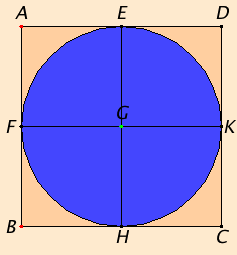# Proposition 8

To inscribe a circle in a given square.

Let ABCD be the given square.

It is required to inscribe a circle in the given square ABCD.

Bisect the straight lines AD and AB at the points E and F respectively. Draw EH through E parallel to either AB or CD, and draw FK through F parallel to either AD or BC.I.34

Therefore each of the figures AK, KB, AH, HD, AG, GC, BG, and GD is a parallelogram, and their opposite sides are evidently equal.

Now, since AD equals AB, and AE is half of AD, and AF half of AB, therefore AE equals AF, so that the opposite sides are also equal, therefore FG equals GE.

Similarly we can prove that each of the straight lines GH and GK equals each of the straight lines FG and GE. Therefore the four straight lines GE, GF, GH, and GK equal one another.

Therefore the circle described with center G and radius one of the straight lines GE, GF, GH, or GK also passes through the remaining points.

And it touches the straight lines AB, BC, CD, and DA, because the angles at E, F, H, and K are right.

III.16

For, if the circle cuts AB, BC, CD, or DA, the straight line drawn at right angles to the diameter of the circle from its end will fall within the circle, which was proved absurd. Therefore the circle described with center G and radius one of the straight lines GE, GF, GH, or GK does not cut the straight lines AB, BC, CD, and DA.

Therefore it touches them, and has been inscribed in the square ABCD.

Therefore a circle has been inscribed in the given square.

Q.E.F.

## Guide

This is a straightforward proposition, one of four in the sequence IV.6 through IV.9 about circles and squares.